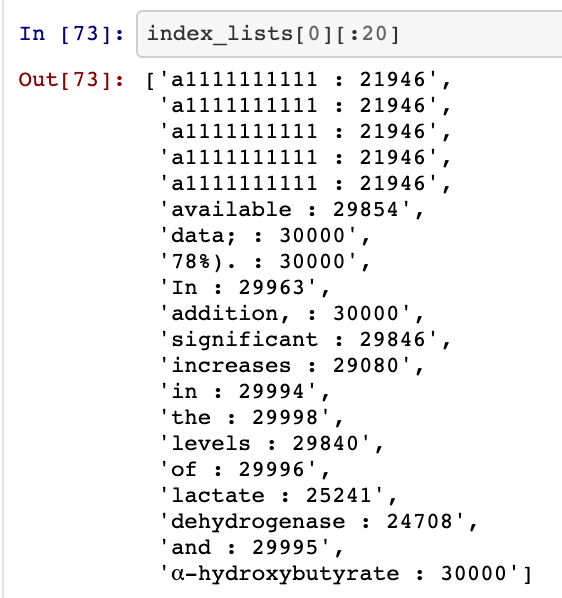# Tokenizing CORD-19 with NLTKExample of tokens mapped to their index positions. Clearly we have problems from the NLTK tokenization such as the “data;” token. I will build on this notebook with more data cleaning in the future to avoid these problems. For now, I think this is a solid tutorial on the basics of building token to index lists.

# NLTK Tokenizer

The NLTK tokenizer is pretty naive compared to things like Byte-Pair encoding or Subword tokenization. However, it gets the job done and is a great baseline for getting started.

`import nltknltk.download('punkt');from nltk.tokenize import word_tokenizetext = "Exploring the CORD19 Dataset"print(word_tokenize(text))# ['Exploring', 'the', 'CORD19', 'Dataset']`
`%%timetext = "Exploring the CORD19 Dataset"word_tokenize(text);# CPU times: user 279 µs, sys: 0ns, total: 279 µs# Wall time: 293 µs`
`%timeword_tokenize(longer_text);# CPU times: user 11.8 ms, sys: 0ns, total: 11.8ms# Wall time: 11.7 ms`

# Top-K Dictionary

• The first step in Tokenization is to build a dictionary that maps from a token to its index in the dictionary, e.g. “hello” → 815
• Loop through the text to count the 30,000 most frequently occurring words
• Assign each of these top 29,999 words an index mapping their top-K rank. If “the” is the 15th most frequently occurring token… it is mapped to index 15.
• All words outside of the top-K will be mapped to 30_000
`%%timefreq_counter = {} # freq short for frequency...for i in df.Sequence: # Sequence is a DataFrame column containing paragraphs     tok_seq = word_tokenize(i)  for tok in tok_seq:    if tok in freq_counter.keys():      freq_counter[tok] += 1    else:      freq_counter[tok] = 1# CPU times: user 5min 5s, sys: 770 ms, total: 5min 6s# Wall time: 5min 7s`
`import operatorsorted_dict = sorted(freq_counter.items(), key=operator.itemgetter(1))sorted_dict[-2:]# [('the', 1567251), (',', 1867586)]`
`print(len(sorted_dict))print(sorted_dict[-30_000:][:5]# 656159# [('scintillation', 41),   ('inositol', 41),   ('Proteintech', 41),   ('stillbirth', 41),   ('colder', 41)]`
`top_K_list = sorted_dict[-29_999:] # 29999 -> 29_999... much more readable`
`top_K_token_to_index_dict = {}top_K_index_to_token_dict = {} # ^ verbose naming, but hopefully more clear for sake of tutorialfor i in range(len(top_K_list)):  top_K_token_index_dict[top_K_list[i]] = counter  top_K_index_token_dict[counter] = top_K_list[i]  counter += 1top_K_index_token_dict[30_000] = "Unknown"`
`import json# Token -> Indextoken_index_dict_write = json.dumps(top_K_token_index_dict)f = open("token_index_dict.json", "w")f.write(token_index_dict_write)f.close()# Index -> Tokenindex_token_dict_write = json.dumps(top_K_index_token_dict)f = open("index_token_dict.json", "w")f.write(index_token_dict_write)f.close()`
`f = open("token_index_dict.json", "r")dict_text = f.readlines()token_index_dict = json.loads(dict_text)`

# Text -> Index Mapping

`def text_to_index(seq, token_index_dict):  idx_lst = []  tok_lst = word_tokenize(seq)  for tok in tok_lst:    if tok not in token_index_dict.keys():      idx_lst.append(30_000)    else:      idx_lst.append(token_index_dict[tok])  return idx_lst`
`sentence = "hello how are you doing"text_to_index(sentence, top_K_token_index_dict)# [30_000, 29675, 29978, 29143, 25834]`

# Build Index Lists

These index lists are the input to our Neural Network

`def build_index_lists(df, text_col_name, text_index_dict):  index_lists = []  for seq in df[text_col_name]:    seq = seq.split(' ')    new_index_list = []    for tok in seq:      if tok in text_index_dict.keys():        new_index_list.append(text_index_dict[tok])      else:        new_index_list.append(30_000)    index_lists.append(new_index_list)  return index_lists`

# Padding or Truncating Sequences to Length k (k=128 in this case)

`def pad_to_length_k(org_index_lists, k):  index_lists = org_index_lists  for seq_list = index_lists:    while (len(seq_list) > k):      seq_list.pop()    while (len(seq_list) < k):      seq_list.append(0)  return index_lists`
`index_lists = pad_to_length_k(index_lists, 128)df["Index_Lists"] = index_listsdf.info()`
`df.to_csv('IdxLists_Pdf_Json_1.csv', index=False)`

# Tokenization Complete: What we need for Downstream Applications

Load the DataFrame with the Index Lists

`from google.colab import filesfiles.upload()`
`df = pd.read_csv('IdxLists_Pdf_Json_1.csv')`
`f = open("token_index_dict.json", "r")dict_text = f.readlines()token_index_dict = json.loads(dict_text)`
`f = open("index_token_dict.json", "r")dict_text = f.readlines()index_token_dict = json.loads(dict_text)`
`git clone https://github.com/CShorten/CORD-19-Mining.git`
`import sys, ossys.path.append(os.getcwd() + '/CORD-19-Mining/')# note, I'll probably group this in a /utils folder soon# so also try    " + '/CORD-19-Mining/utils/') "   if it doesn't workfrom tokenization import text_to_index, index_to_text`
`text_to_index("hello how are you", token_index_dict)`

# Conclusion

This notebook has shown you how to tokenize text stored as rows in DataFrame column with the NLTK tokenizer. This was done by assigning indexes based on the top 30K most frequently occurring words. I expect this tokenization to be too naive for applications like information retrieval, question answering, or summarization. However, I think this is a solid place to get started and at least we have the index lists to build the models and get that half of the pipeline working properly.

--

--